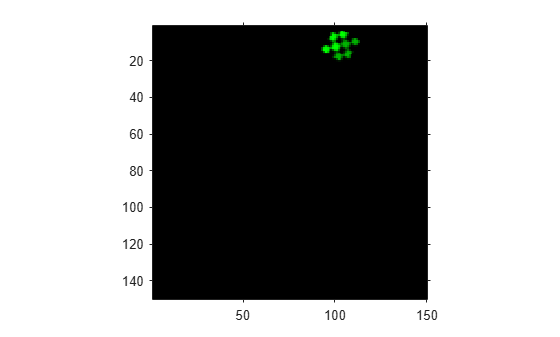# Create Composite 2-D Affine Transformations

This example shows how to create a composite of 2-D translation and rotation transformations. The order of the transformation matters, so there are two approaches to creating a composition:

1) Create a matrix that represents the individual transformations, then create the composite transformation by multiplying the matrices together, and finally store the transformation matrix as an `affinetform2d` object. This approach has the most flexibility because you can use all types of affine transformations including shear, reflection, anisotropic scaling, and other composite affine transformations. You can also control the order of transformations through the order of the matrix multiplication.

2) Create a `simtform2d`, `rigidtform2d`, or `transltform2d` geometric object. This approach makes it easy to create a composite transformation when you know the scale factor, rotation angle, and translation distance of a nonreflective similarity transformation. However, the geometric transformation objects always perform the relevant transformations in a fixed order: scaling first, then rotation, then translation. If you need to perform the transformations in a different order, then you must use matrices and create an affine transformation object.

This example demonstrates both approaches for a rigid transformation consisting of translation and rotation.

Create a checkerboard image that will undergo transformation. Also create a spatial reference object for the image.

```cb = checkerboard(4,2); cb_ref = imref2d(size(cb));```

To illustrate the spatial position of the image, create a flat background image. Overlay the checkerboard over the background, highlighting the position of the checkerboard in green.

```background = zeros(150); imshowpair(cb,cb_ref,background,imref2d(size(background)))```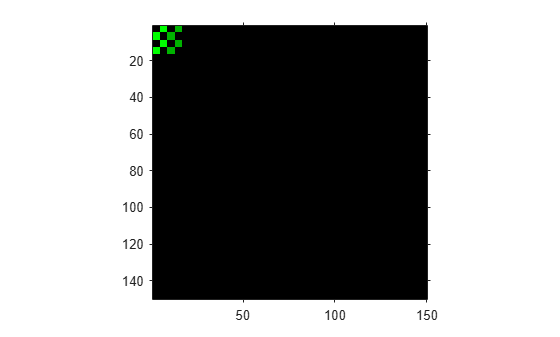Create a translation matrix that shifts an image horizontally and vertically.

```tx =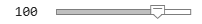100; ty =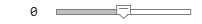0; T = [1 0 tx; 0 1 ty; 0 0 1]```
```T = 3×3 1 0 100 0 1 0 0 0 1 ```

Create a rotation matrix that rotates an image clockwise about the origin.

```theta =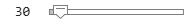30; R = [cosd(theta) -sind(theta) 0; sind(theta) cosd(theta) 0; 0 0 1]```
```R = 3×3 0.8660 -0.5000 0 0.5000 0.8660 0 0 0 1.0000 ```

### Rotation Followed by Translation using Matrices

Perform rotation first and translation second. Using the premultiply matrix convention, the translation matrix `T` is on the left and the rotation matrix `R` is on the right.

`TR = T*R`
```TR = 3×3 0.8660 -0.5000 100.0000 0.5000 0.8660 0 0 0 1.0000 ```

Store the composite transformation as an affine transformation, then warp the original checkerboard image. Display the result with spatial referencing.

```tform_tr = affinetform2d(TR); [out,out_ref] = imwarp(cb,cb_ref,tform_tr); imshowpair(out,out_ref,background,imref2d(size(background)))```### Translation Followed by Rotation using Matrices

Reverse the order of the transformations: perform translation first and rotation second. Using the premultiply matrix convention, the rotation matrix `R` is on the left and the translation matrix `T` is on the right.

`RT = R*T`
```RT = 3×3 0.8660 -0.5000 86.6025 0.5000 0.8660 50.0000 0 0 1.0000 ```

Store the composite transformation as an affine transformation, then warp the original checkerboard image. Display the result with spatial referencing. Notice how the spatial position of the transformed image is different than when translation was followed by rotation.

```tform_rt = affinetform2d(RT); [out,out_ref] = imwarp(cb,cb_ref,tform_rt); imshowpair(out,out_ref,background,imref2d(size(background)))```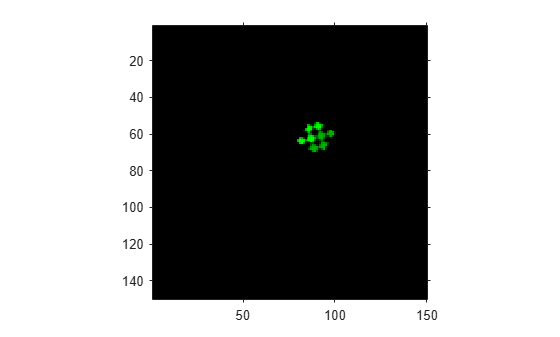### Rotation Followed by Translation using Rigid Transformation Object

Create a rigid geometric transformation object with the rotation angle and translation distance that were specified earlier in the example.

`tform_rigid = rigidtform2d(theta,[tx ty]);`

View the geometric transformation matrix by querying the `A` property. The matrix is equal to the matrix `TR` as expected, because a rigid transformation object performs rotation before translation.

`tform_rigid.A`
```ans = 3×3 0.8660 -0.5000 100.0000 0.5000 0.8660 0 0 0 1.0000 ```

Confirm the order of operations by warping the test image and displaying the result with spatial referencing. The result is identical to the result obtained through matrix multiplication.

```[out,out_ref] = imwarp(cb,cb_ref,tform_rigid); imshowpair(out,out_ref,background,imref2d(size(background)))```